# 1. 非监督学习

• unsupervised learnings 非监督学习
• clusteing 聚类
• dimensionality reduction 降维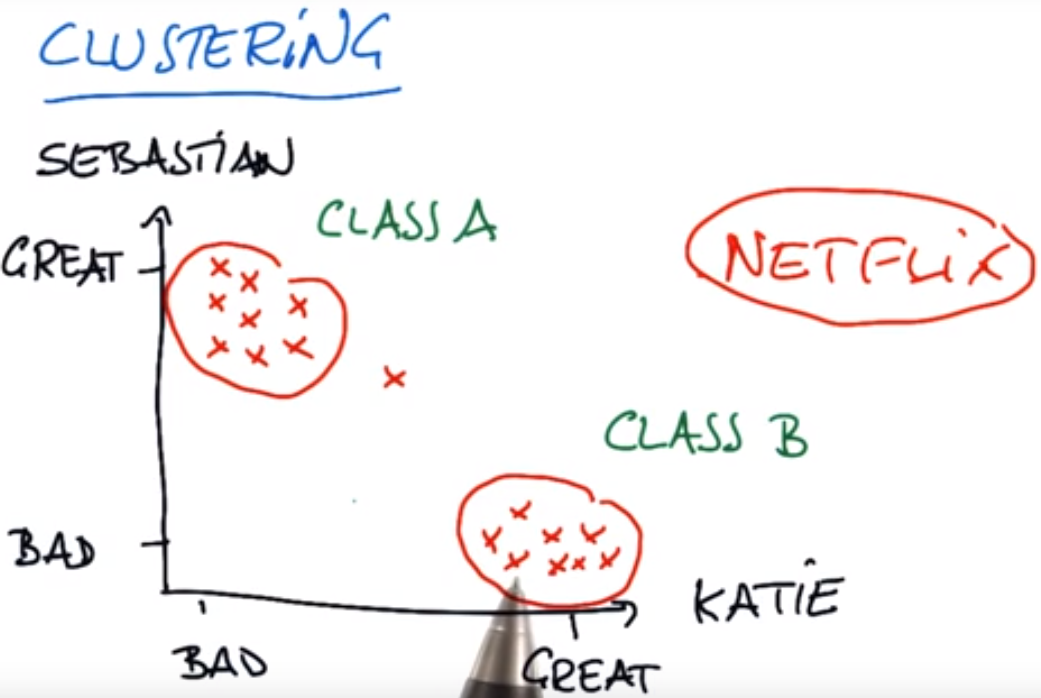# 4. 将点与聚类匹配

• 第一步先分配，即随机分配聚类中心点
• 第二步，再优化，利用下面的最短距离和方法，不断移动聚类中心点
• 绿色点假设是聚类中心，判断哪些红色点与中心点1距离，比中心点2的距离更短：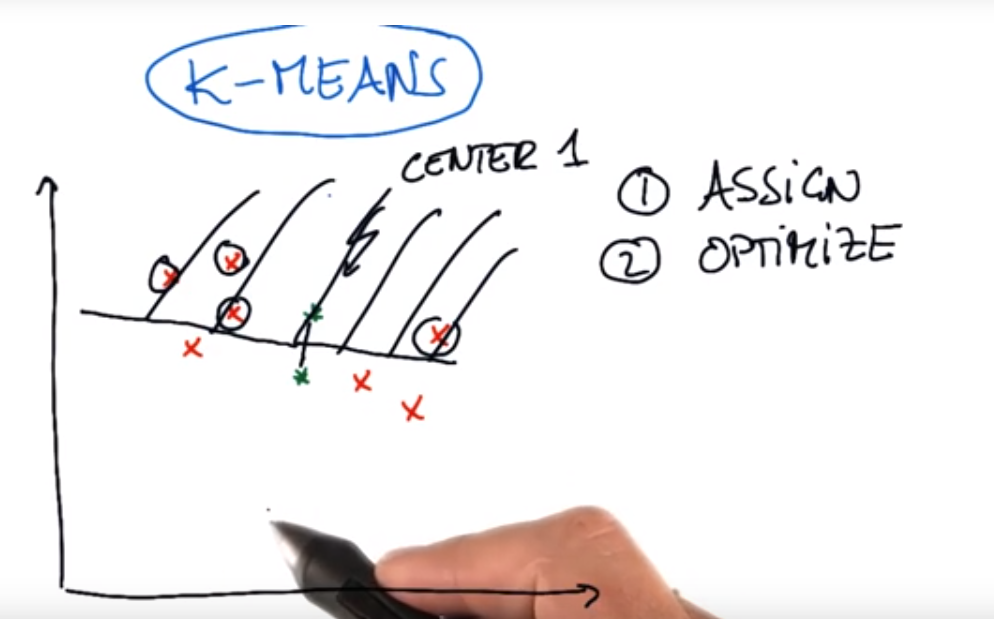# 5. 优化中心(橡皮筋)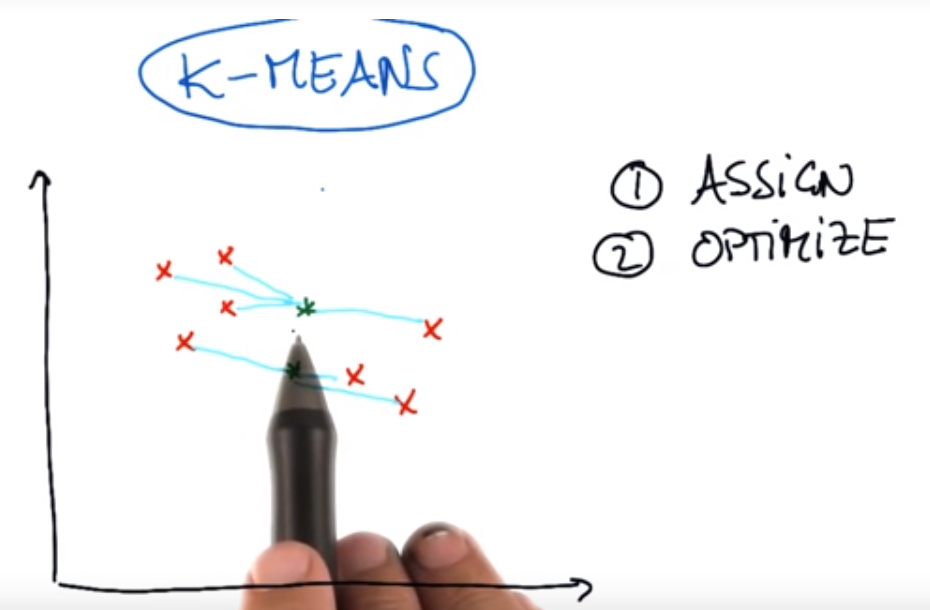# 6. 移动中心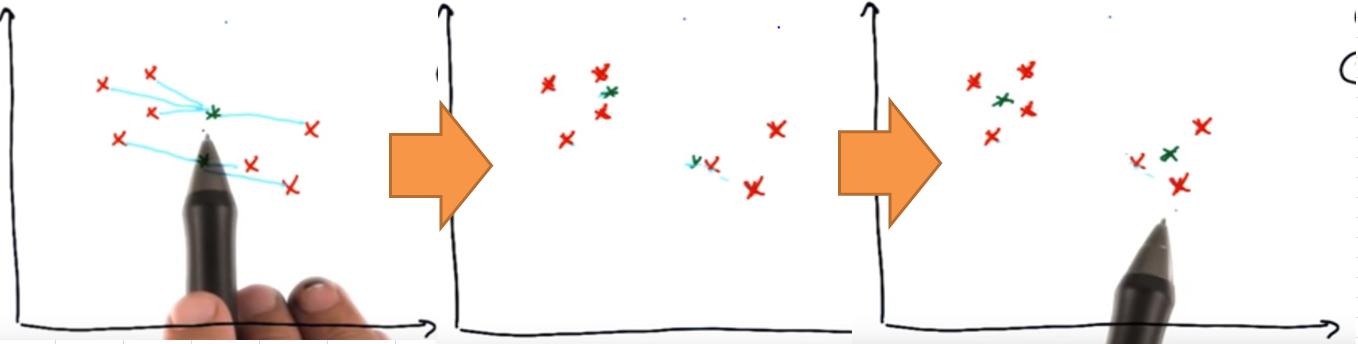# 12. Sklearn中的聚类

``````class sklearn.cluster.KMeans(n_clusters=8, init=’k-means++’, n_init=10, max_iter=300, tol=0.0001, precompute_distances=’auto’, verbose=0, random_state=None, copy_x=True, n_jobs=1, algorithm=’auto’)
``````
• `n_clusters`:聚类数量，一般要根据实际情况设置
• `max_iter=300`:移动的最大次数，一般在300次之前就已经找到最好的中心了

• 示例如下:
``````>>> from sklearn.cluster import KMeans
>>> import numpy as np
>>> X = np.array([[1, 2], [1, 4], [1, 0],
...               [4, 2], [4, 4], [4, 0]])

#  2 个聚类，对X数据进行训练
>>> kmeans = KMeans(n_clusters=2, random_state=0).fit(X)

# 上面训练出来的标签
>>> kmeans.labels_
array([0, 0, 0, 1, 1, 1], dtype=int32)

# 预测新的数据
>>> kmeans.predict([[0, 0], [4, 4]])
array([0, 1], dtype=int32)

# 2个聚类的中心点
>>> kmeans.cluster_centers_
array([[ 1.,  2.],
[ 4.,  2.]])
``````

# 15. 反直觉的聚类

• 图1，浅蓝色是期望的聚类中心，但是可能会变成深绿色的聚类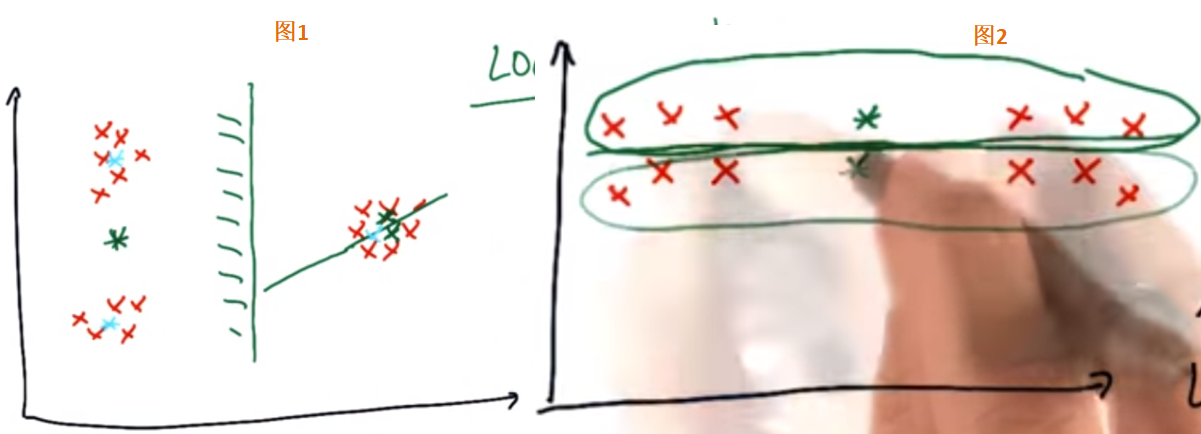# 18. K-均值聚类迷你项目 - 部署聚类

``````from sklearn.cluster import KMeans

kmeans = KMeans(n_clusters=2, random_state=0).fit(finance_features)
pred = kmeans.labels_
``````
• 图形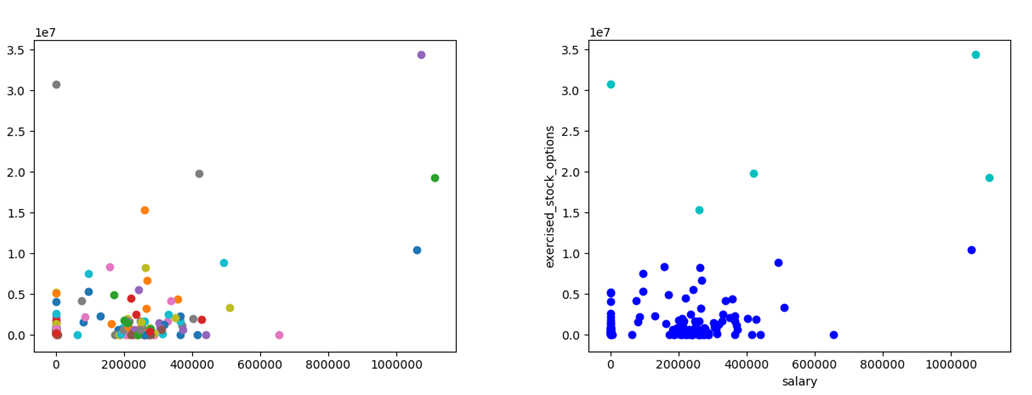# 19. 使用 3 个特征聚类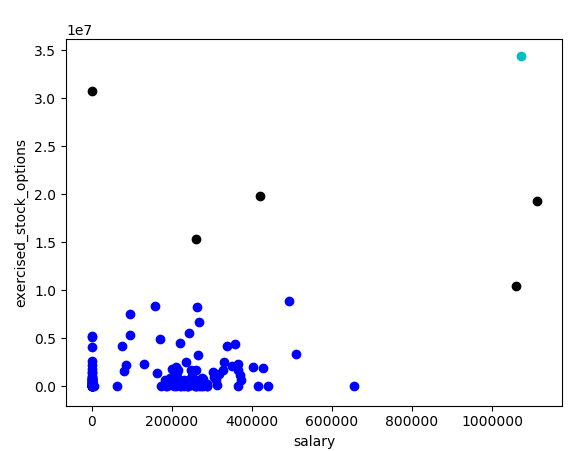# 22. 股票期权范围

“exercised_stock_options”的最大值和最小值（忽略“NaN”）

``````data_find = []
for k,v in data_dict.items():
value = v["exercised_stock_options"]
if value != "NaN":
data_find.append(value)

data_find.sort()

print data_find
print data_find[-1]
``````

``````3285
34348384
``````

``````477
1111258
``````